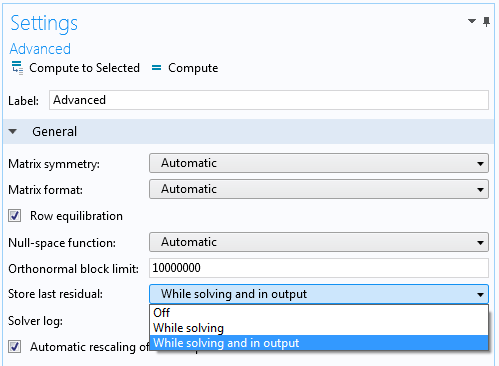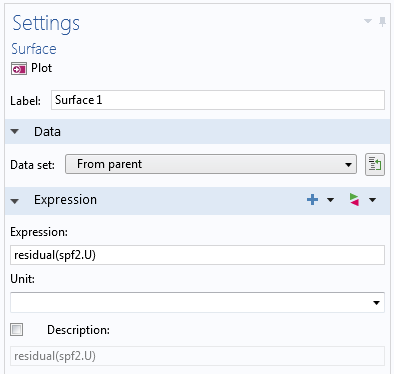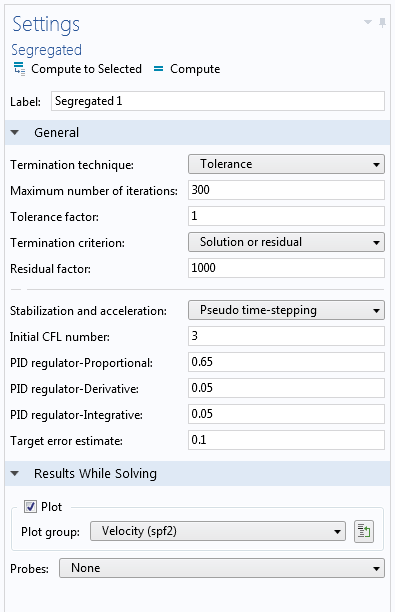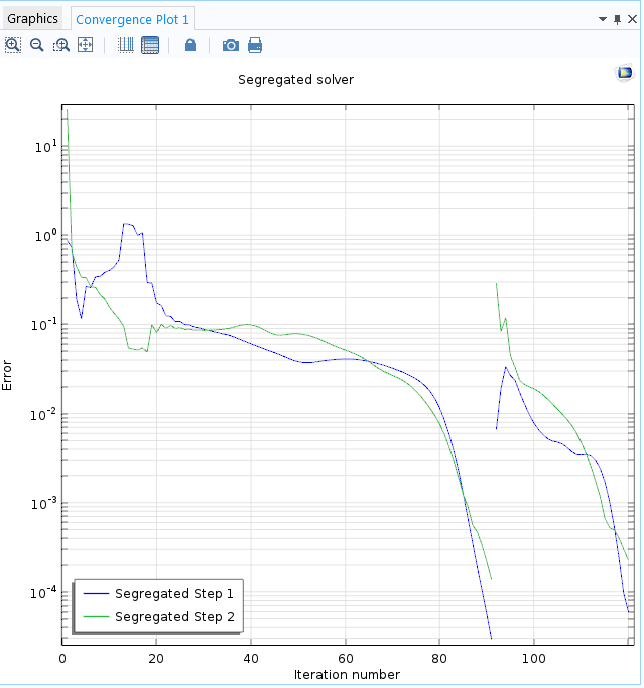# Plotting the Algebraic Residual to Study Model Convergence

April 27, 2016

You can use the `residual` operator, new with COMSOL Multiphysics version 5.2, to evaluate and plot your model’s algebraic residual in order to troubleshoot convergence issues. This blog post demonstrates the use of the ` residual` operator for visualizing and understanding the convergence properties of a turbulent flow simulation.

### What Is the Algebraic Residual?

When solving a finite element model, it is important to know how accurate the results are. Depending on the mesh (the discretization) and the nature of the equations for which you solve, the algebraic residual is only one of several error sources. The following sources contribute to the computation error:

• The truncation error (also called the Galerkin error for the finite element method).
• The quadrature error made by using numerical methods to approximate the finite element integrals.
• The geometrical approximation error made by representing the actual geometry by a polynomial representation, which is a sort of integration error for elements adjacent to or on a curved boundary.
• The algebraic error obtained by terminating the solvers prematurely (or by using a sloppy tolerance). This is the error that you can access using the `residual` operator.

Normally, the algebraic error is (and should be) much smaller than the truncation error. However, if you run into convergence issues, the algebraic residual is not small and can reveal where the cause of the issue resides in your model.

Contrary to the related information that COMSOL Multiphysics already provides, such as convergence numbers, which are scaled global numbers, the `residual` operator provides an unscaled residual value for each variable in a model. It also shows a spatial distribution of the residual for that quantity. The residual is the latest-computed residual vector from the finite element discretization, but interpreted as a continuous spatial field quantity. The spatial distribution helps you pinpoint where in the modeling domain the residual is relatively large and therefore will hamper convergence of the solution. Possible problems in the model could be:

• Insufficient mesh resolution in that part of the modeling domain
• Sharp corners in the geometry
• Inappropriate or incompatible boundary conditions

Let’s see exactly how to plot and evaluate the algebraic residual in your model with the `residual` operator.

### How to Add the Algebraic Residual to a Model

The `residual` operator is available for stationary and time-dependent simulations. To add the `residual` operator to the model, you need to activate it using a setting in the Advanced subnode to a stationary or time-dependent solver. By default, the Store latest residual setting is ` Off`. To compute the residual, choose While solving, or to compute it and store it in the output for postprocessing, choose While solving and in output. Storing the residual in the output so that you can use it in plots and for general postprocessing requires additional memory resources, but this is not necessary if you want to plot the residual while solving only.Settings for specifying the storage of the last residual values.

### Using the `Residual` Operator in COMSOL Multiphysics: A Fluid Dynamics Example

To demonstrate how the `residual` operator can provide insight into how numerical disturbances can enter and propagate in a finite element model, consider the Turbulent Flow Over a Backward Facing Step example model from the Application Gallery. This example uses the k-ɛ turbulence model. The model features a classic 2D geometry of a backward facing step with a corner that causes a recirculation zone in a turbulent fluid flow simulation.

This version of the model includes plotting the residual in the momentum equations (the velocity field) while solving. To do so, a Velocity plot group contains a Surface plot node with the expression `residual(spf2.U)`, where ` spf2.U` is the velocity magnitude.Part of the Surface plot node’s Settings window, with the `residual` operator as the expression to plot.

The updates are computed for each segregated iteration. In the Segregated solver node, pseudo time stepping is used for stabilization and acceleration, and the Velocity plot group is selected as the plot to show while solving. For such a plot, it is sufficient to set the Store last residual feature to the While solving option. Also note that if you choose Residual as the Termination criterion for the segregated solver, then you get the same residual values as those provided by the `residual` operator, which determine the convergence. However, for the default solution or residual criterion, the ` residual` operator also provides important information.The Segregated solver settings with the plot of the residual, specified under Results While Solving.

This example is a nice illustration of what happens when you solve transport problems with some sort of stationary approach — in this case, pseudo time stepping. The residual caused by the “backstep disturbance” needs to be transported out of the region. The residual does not drop until the “disturbances” have been transported out of the channel and the simulation can finish. The screenshots below show how the area where the residual is relatively large moves from the inlet area toward the outlet.

The residual for the velocity field at the beginning and toward the end of the solution.

The convergence plot confirms that the initially sluggish convergence changes to a quick convergence in just a few iterations once the large residual has disappeared. This behavior is consistent with the fact that perturbations and errors evolve according to the approximated equation — in this case, an approximation of time-dependent, convection-dominated flow equations. The errors therefore need time, which corresponds to iterations for pseudo time stepping, to be transported out of the domain.The convergence plot for the Turbulent Flow Over a Backward Facing Step tutorial model. Notice the fast convergence toward the end.

By plotting and evaluating the algebraic residual, you can troubleshoot models that do not converge or converge slowly, so that you can find your simulation results as quickly and efficiently as possible.

### Further Resources

#### Categories##### Michael Rembe
August 19, 2016

Hello Magnus,

thank you very much for your explanations. The residual helps to evaluate the solution and to get an idea about convergence problems.

If you miss the residual, COMSOL52a doesn’t save any residuals in the case you cancel simulation by a stop condition (with saving results). In this case you get the residual by results during simulation.

Best regards##### Ulrik Thisted
September 20, 2016

Hello,

This feature sound very useful and I have missed it in the past.

Best regards
Ulrik##### Milan Patel
December 1, 2016

Me too! This would be great.

EXPLORE COMSOL BLOG